# Z9–I–1

In all nine fields of given shape to be filled natural numbers so that:

• each of the numbers 2, 4, 6, and 8 is used at least once,
• four of the inner square boxes containing the products of the numbers of adjacent cells of the outer square,
• in the circle is the sum of the numbers of adjacent cells of the inner square.

Find out what the smallest and the largest number that can be written in a circle.

a =  0
b =  0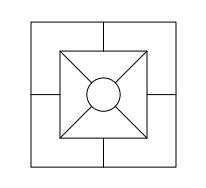Did you find an error or inaccuracy? Feel free to write us. Thank you!#### You need to know the following knowledge to solve this word math problem:

We encourage you to watch this tutorial video on this math problem:

## Related math problems and questions:

• Octahedron - sumOn each wall of a regular octahedron is written one of the numbers 1, 2, 3, 4, 5, 6, 7 and 8, wherein on different sides are different numbers. For each wall John make the sum of the numbers written of three adjacent walls. Thus got eight sums, which also
• Z9–I–4 MO 2017Numbers 1, 2, 3, 4, 5, 6, 7, 8 and 9 were prepared for a train journey with three wagons. They wanted to sit out so that three numbers were seated in each carriage and the largest of each of the three was equal to the sum of the remaining two. The conduct
• Six-digit primesFind all six-digit prime numbers that contain each one of digits 1,2,4,5,7, and 8 just once. How many are they?
• DecideThe rectangle is divided into seven fields. On each box is to write just one of the numbers 1, 2 and 3. Mirek argue that it can be done so that the sum of the two numbers written next to each other was always different. Zuzana (Susan) instead argue that i
• Z9-I-4Kate thought a five-digit integer. She wrote the sum of this number and its half at the first line to the workbook. On the second line wrote a total of this number and its one fifth. On the third row, she wrote a sum of this number and its one nines. Fina
• Number train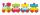The numbers 1,2,3,4,5,6,7,8 and 9 traveled by train. The train had three cars and each was carrying just three numbers. No. 1 rode in the first carriage, and in the last carriage was all odd numbers. The conductor calculated sum of the numbers in the firs
• Pyramid Z8–I–6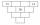Each brick of the pyramid contains one number. Whenever possible, the number in each brick is the lowest common multiple of two numbers of bricks lying directly above it. May that number be in the lowest brick? Determine all possibilities.
• Square gridSquare grid consists of a square with sides of length 1 cm. Draw in it at least three different patterns such that each had a content of 6 cm2 and circumference 12 cm and that their sides is in square grid.
• MO Z6-6-1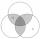Write integers greater than 1 to the blanks in the following figure so that each darker box was a product of the numbers in the neighboring lighter boxes. What number is in the middlebox?
• Coloured numbersMussel wrote four different natural numbers with coloured markers: red, blue, green and yellow. When the red number divides by blue, it gets the green number as an incomplete proportion, and yellow represents the remainder after this division. When it div
• Star equationWrite digits instead of stars so that the sum of the written digits is odd and is true equality: 42 · ∗8 = 2 ∗∗∗
• Air draftThe numbers 1,2,3,4,5 are written on five tickets on the table. Air draft randomly shuffled the tickets and composed a 5-digit number from them. What is the probability that he passed: and, the largest possible number b, the smallest possible number c, a
• Bridge cards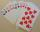How many bridge hands are possible containing 4 spades,6 diamonds, 1 club, and 2 hearts?
• Square ABCDConstruct a square ABCD with cente S [3,2] and the side a = 4 cm. Point A lies on the x-axis. Construct square image in the displacement given by oriented segment SS'; S` [-1 - 4].
• Endless lego setThe endless lego set contains only 6, 9, 20 kilograms blocks that can no longer be polished or broken. The workers took them to the gym and immediately started building different buildings. And of course, they wrote down how much the building weighed. The
• Outer contact of circles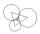Construct a circle k1 (S1; 1.5 cm), k2 (S2; 2 cm), and K3 (S3; 2.5 cm) so that they are always two outer contacts. Calculate the perimeter of the triangle S1S2S3.
• Fluid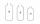We have vessels containing 7 liters, 5 liters and 2 liters. Largest container is filled with fluid the others empty. Can you only by pouring get 5 liters and two 1 liter of fluid? How many pouring is needed?